Keris
 Home Quick Tour    Part 1: Basics    Part 2: Extensibiliy    Part 3: Classes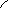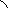Quick Tour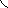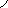Part I: Basics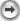This page will give you a brief introduction to the syntax and semantics of Keris. It will explain how to develop and evolve modules, the basic building blocks of software written in Keris. The example code is complete and can be compiled using the Keris compiler keco. You can download a package containing all example files here. A file Module.keris is compiled with the following command: keco Module.keris.

1  Defining Modules
In Keris, modules are the basic top-level abstractions for software components supporting separate compilation as well as function and type abstraction in an extensible fashion. Here is a small module MATHS that encapsulates a few arithmetical functions for floating-point numbers.

 ```module MATHS { float abs(float x) { return (x < 0) ? -x : x; } float sqr(float x) { return x * x; } float sqrt(float x) { float guess = 1.0f; while (!goodEnough(guess, x)) guess = (guess + x / guess) / 2.0f; return guess; } private boolean goodEnough(float guess, float x) { return MATHS.abs(sqr(guess) - x) < 0.01f; } } ```

MATHS exports all public functions; private functions cannot be accessed from clients of MATHS. Functions without modifiers are public by default. The example also shows that functions of the own module can be accessed either in unqualified form, or by qualifying the function name with the module name.

We can now write a module that makes use of the functionality provided by module MATHS. In the following example we implement a function distance which calculates the geometric distance between two points in a 2-dimensional space. The dependency on module MATHS is expressed by explicitly stating MATHS in the requirement clause of our new module GEO. In addition to MATHS, we have a second dependency on a module INOUT.

 ```module GEO requires MATHS, INOUT { float distance(float x1, float y1, float x2, float y2) { return MATHS.sqrt(MATHS.sqr(x1 - x2) + MATHS.sqr(y1 - y2)); } void print(float x, float y) { INOUT.write("(" + x + ", " + y + ")"); } } ```

Again, we access members of required modules by qualifying their name with the corresponding module name. Since this can be cumbersome, Keris provides means to import members of other modules, so that they can be accessed without explicit qualification. Here is an alternative implementation that imports all members of MATHS into the scope of GEO.

 ```module GEO requires MATHS, INOUT { import MATHS.*; float distance(float x1, float y1, float x2, float y2) { return sqrt(sqr(x1 - x2) + sqr(y1 - y2)); } void print(float x, float y) { INOUT.write("(" + x + ", " + y + ")"); } } ```

It remains to show a specification of module INOUT. We do this by defining a module interface that specifies the signature of this module. Such a module interface does not contain code, it only specifies the types of members provided by a concrete implementation of this module.

 ```module interface INOUT { String read(); void write(String str); } ```

We will now define a module CONSOLE that implements this interface and thus is a possible candidate for being used together with module GEO which requires an implementation of INOUT.

 ```module CONSOLE implements INOUT { String read() { try { return new java.io.DataInputStream(System.in).readLine(); } catch (java.io.IOException e) { return null; } } void write(String str) { System.out.print(str); } } ```

This module implements the functions read and write by forwarding the calls to appropriate methods of the standard Java API for text in- and output on a terminal. It is of course possible to have alternative implementations for INOUT, as well as one module implementing multiple module interfaces. Please note that module GEO and MATHS do not explicitly implement a module interface. This is not strictly necessary since every module declaration implicitly defines a module interface of the same name with all public members. Nevertheless, the separation of module implementations from interfaces is important for the following reasons:

• It enables separate compilation of recursively dependent modules,
• it is a facility for hiding concrete representations of module members, and
• it can be used as a vehicle for presenting different views of a single module implementation.

Keris distinguishes between modules and module instances. A module can be seen as a template for multiple module instances of the same structure and type. This distinction is necessary to allow a module to be deployed multiple times within a software system.

In Keris, modules are composed by aggregation; i.e. a Keris module may define a set of submodules (submodule instances would propably be the more exact term). It can access both required modules and submodules in its scope. The following example code implements a module GEOAPP which links modules MATHS and GEO by declaring both to be submodules. Submodule declarations start with the keyword module followed by the name of the module implementation.

 ```module GEOAPP requires INOUT { module GEO; module MATHS; float readFloat() { while (true) try { return Float.parseFloat(INOUT.read()); } catch (NumberFormatException e) {} } float pointDistance() { INOUT.write("x1 = "); float x1 = readFloat(); INOUT.write("y1 = "); float y1 = readFloat(); INOUT.write("x2 = "); float x2 = readFloat(); INOUT.write("y2 = "); float y2 = readFloat(); return GEO.distance(x1, y1, x2, y2); } } ```

A module M can only aggregate a submodule S, if all modules required by S are accessible in the scope of M. A module N is accessible in the scope of M if N refers to M directly, or if N is required by M, or if N is a submodule of M. Therefore, the previous module is well-formed, since submodule MATHS has no context dependencies, and submodule GEO requires the modules MATHS and INOUT which are both accessible in GEOAPP.

Note that we do not refer to module MATHS directly in the body of GEOAPP. We introduced this submodule only to satisfy the requirements of submodule GEO. Alternatively to this aggregation, we could have also added MATHS to the requirements.

We now assemble a completely self-contained module MAIN (i.e. a module without context dependencies) which links module GEOAPP with an implementation of module INOUT.

 ```module MAIN { module GEOAPP; module CONSOLE; void main(String[] args) { CONSOLE.write("distance = " + GEOAPP.pointDistance() + "\n"); } } ```

Modules without context dependencies which define a main method of the following signature: void main(String[] args), are executable. Modules get executed like Java classes. For running our MAIN module, one simply has to execute the command: java MAIN.